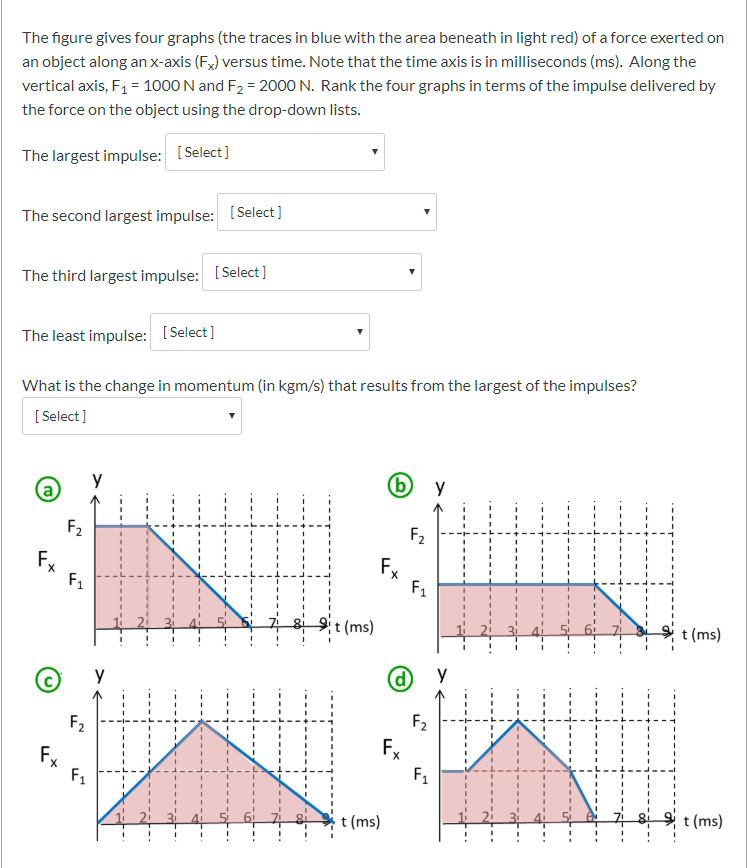# Question Options from drop down for 1-4: a,b,c,d Options for #5: 7.5, 8, 8.5, 9, 9.5, 10 The figure gives four graphs (the traces in blue with the area beneath in light red) of a force exerted on an object along an x-axis (Fx) versus time. Note that the time axis is in milliseconds (ms). Along the vertical axis, F1 = 1000 N and F2 = 2000 N. Rank the four graphs in terms of the impulse delivered by the force on the object using the drop-down lists. The largest impulse: [Select] The second largest impulse: (Select] The third largest impulse: [Select] The least impulse: [Select] What is the change in momentum (in kgm/s) that results from the largest of the impulses? [Select] 11 3 4 5 6 7 8 9t(ms) 4 44 t (ms) (d) y 1 2 3 4 5 6 7 8 t (ms) 11 2 3 4 5 7 8 9t(ms)Options from drop down for 1-4: a,b,c,d

Options for #5: 7.5, 8, 8.5, 9, 9.5, 10

Transcribed Image Text: The figure gives four graphs (the traces in blue with the area beneath in light red) of a force exerted on an object along an x-axis (Fx) versus time. Note that the time axis is in milliseconds (ms). Along the vertical axis, F1 = 1000 N and F2 = 2000 N. Rank the four graphs in terms of the impulse delivered by the force on the object using the drop-down lists. The largest impulse: [Select] The second largest impulse: (Select] The third largest impulse: [Select] The least impulse: [Select] What is the change in momentum (in kgm/s) that results from the largest of the impulses? [Select] 11 3 4 5 6 7 8 9t(ms) 4 44 t (ms) (d) y 1 2 3 4 5 6 7 8 t (ms) 11 2 3 4 5 7 8 9t(ms)
More
Transcribed Image Text: The figure gives four graphs (the traces in blue with the area beneath in light red) of a force exerted on an object along an x-axis (Fx) versus time. Note that the time axis is in milliseconds (ms). Along the vertical axis, F1 = 1000 N and F2 = 2000 N. Rank the four graphs in terms of the impulse delivered by the force on the object using the drop-down lists. The largest impulse: [Select] The second largest impulse: (Select] The third largest impulse: [Select] The least impulse: [Select] What is the change in momentum (in kgm/s) that results from the largest of the impulses? [Select] 11 3 4 5 6 7 8 9t(ms) 4 44 t (ms) (d) y 1 2 3 4 5 6 7 8 t (ms) 11 2 3 4 5 7 8 9t(ms)